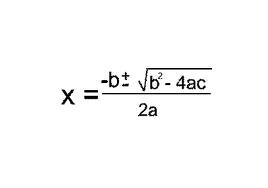# how do you do factorise quadratic equations ?

Help :(

Posted Wed 3rd April, 2013 @ 11:25 by Cara

Take the equation

x(2) + 7x + 12 = 0                  (2) squared

You need to find two numbers that add to make 7 and multiply to make twelve.

3 and 4. 3 * 4 = 12, 3 + 4 =7.

(x + 3)(x + 4) = 0

To solve it you then switch the signs so: x = -3 and x = -4

Substitute them into the equation to check that they work:

-3(2) + 7*-3 + 12 = 0

-4(2) + 7*-4 + 12 = 0

They both work :)

Try another:

x(2) + 9x + 20 = 0

4 + 5 = 9, 4 * 5 = 20

(x + 4)(x + 5) = 0

switch the signs: x = -4, x = -5

If you are unable to find numbers that work however you have to do the quadratic equation:

where  ax(2) + bx + c =0 you just sub in the numbers to the equation below.Hope I have been of assistance to you :)

Answered Wed 3rd April, 2013 @ 11:56 by CoolKid

If there is a coefficient in front of the x2    :    e.g.    2x2 + 7x + 3

You multiply the number in front of the x2 and the constant at the end:   2 x 3 = 6

You then need to find two numbers that multiply to make 6, and add to make 7 (the number in front of x).

1 x 6 = 6     ,      1 + 6 = 7

Instead of writing 2x2 + 7x + 3   ,  you write    2x2 + 1x + 6x + 3

(Exchanging the 7x for 1x + 6x)

2x2 + 1x + 6x + 3

See what is common to the first two terms  2x+ 1x   .......     it's x

Put x outside the bracket:   x(2x + 1)       x goes into 2x2    , 2x times     &     x goes into 1x,   1 time

Do the same for the other two terms  6x + 3    :     3  is common to both terms

Put 3 outside the bracket:   3(2x+1)

Add   x(2x + 1) + 3(2x + 1)           (note that the two brackets should work out to be the same)

Take the values outside the brackets    (x + 3)   and multiply by the value inside the brackets (2x + 1)

Final answer = (x + 3)(2x + 1)

Answered Thu 18th April, 2013 @ 20:49 by Charlotte